••••••••# Supersonic obstacle detection and avoidance.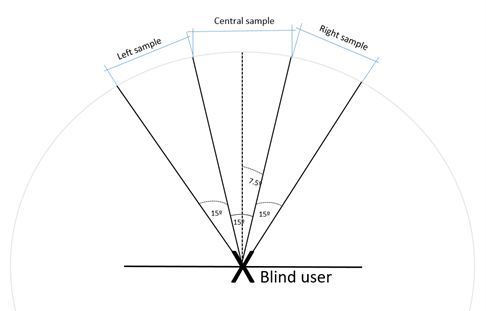Figure 1: Operation principle of the servo-sonar system.

Continuous frontal and left and right lateral detection via a repeated rotation of a supersonic sensor using a servo mechanism. The servo mechanism rotates the supersonic sensor 15 degrees to the left and to the right of the frontal position creating a 45 degrees viewing angle along the user path.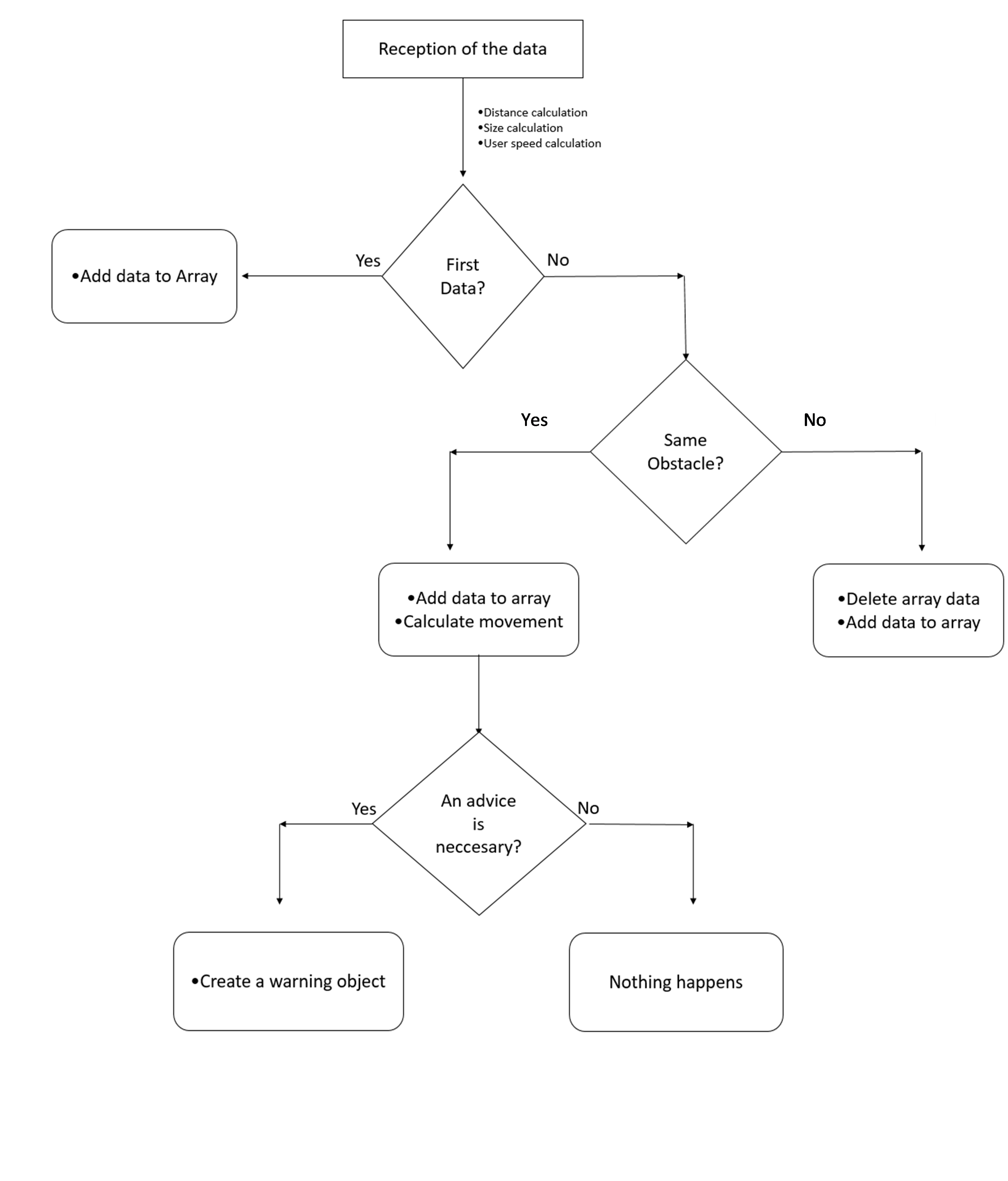Figure 2: Flowchart of the obstacle detection algorithm.

The smartphone navigation application is continuously processing the detection data reported by the supersonic sensor in order to calculate the user distance from the obstacle, user speed and obstacle width, so as to promptly warn the user with proper avoidance instructions.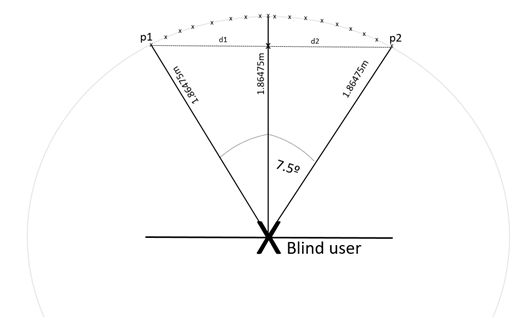Figure 3: Obstacle width calculation.

In order to explain the procedure, the simplest case is assumed, being the lateral samples not having detected any obstacle. The exact position in which the object is found is not known, because if the sensor covers an angle of 15 degrees, mathematically, there are infinite points at 1.86475 meters (the example distance) in which the obstacle can be found. Knowing the arc formed by an angle of 15 degrees in a circle of radius r = 1.86475m, the distance 0.48679m between the leftmost point (p1) and the rightmost point (p2) can be easily calculated by applying some simple trigonometric formulas. It can be assumed that the object has a width of 0.48679m, and that to the left and right of that distance there is no object, therefore the user could avoid the obstacle simply by moving that distance to the left or to the right. The procedure if the lateral samples have detected an obstacle is similar. In that case the sensor will be covering an angle of 30 or 45 degrees.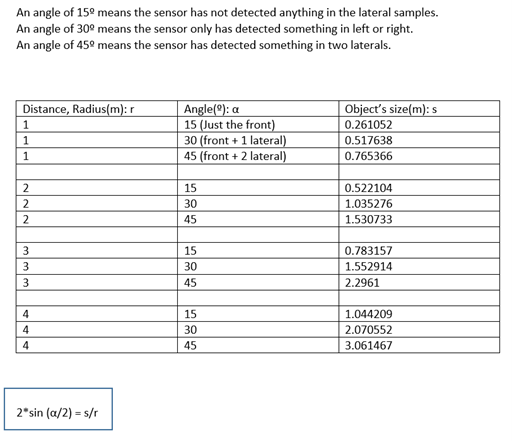Figure 4: Examples of calculated object size for different detection distances and angles.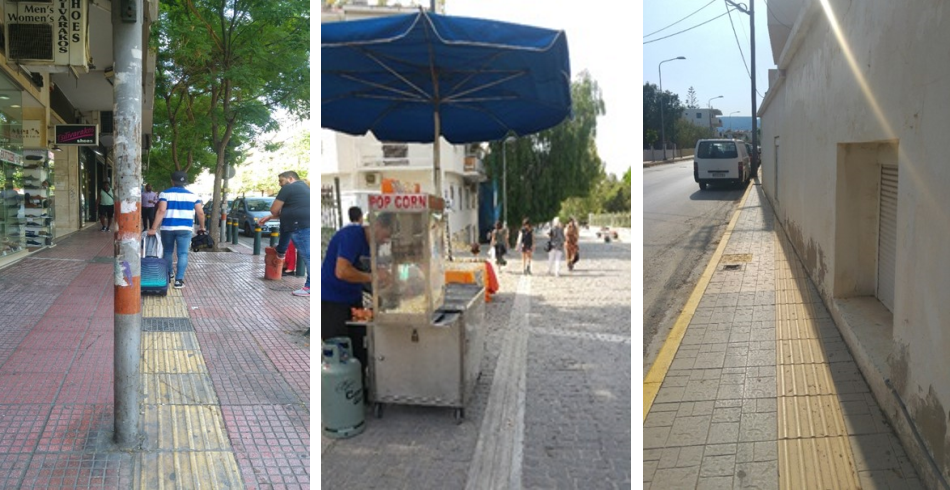Figure 5: Examples of small and medium width objects.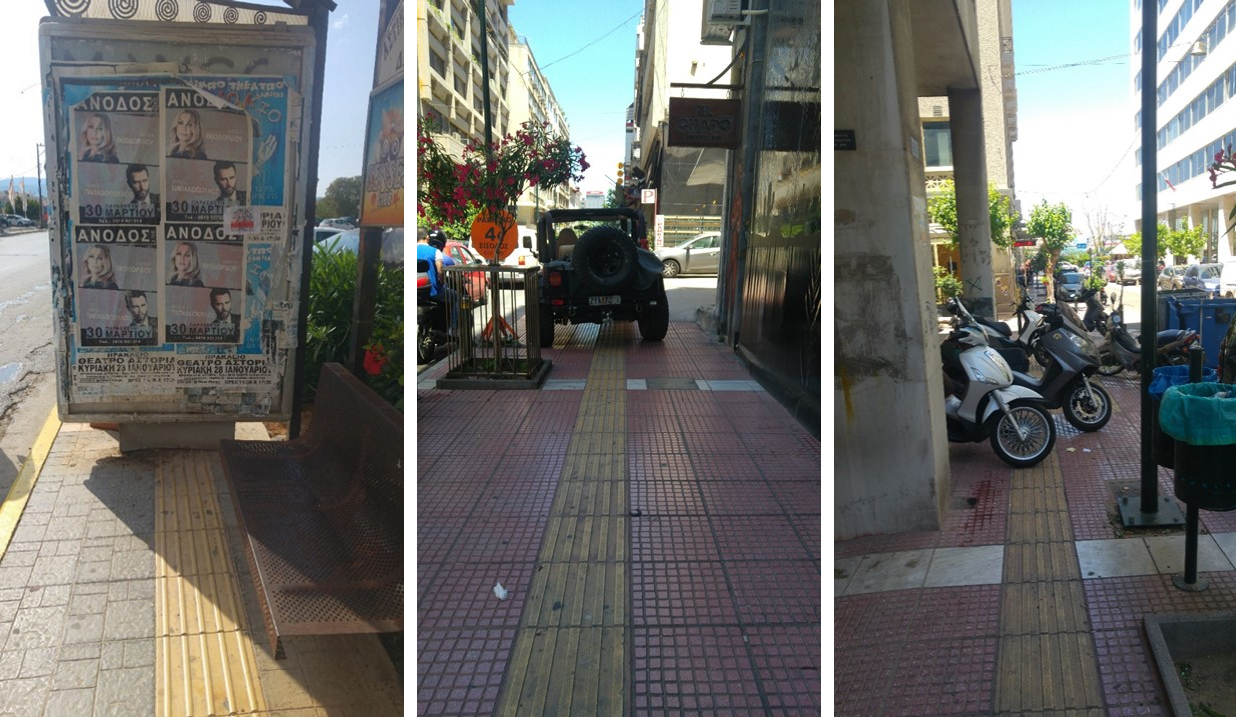Figure 6: Examples of large width objects.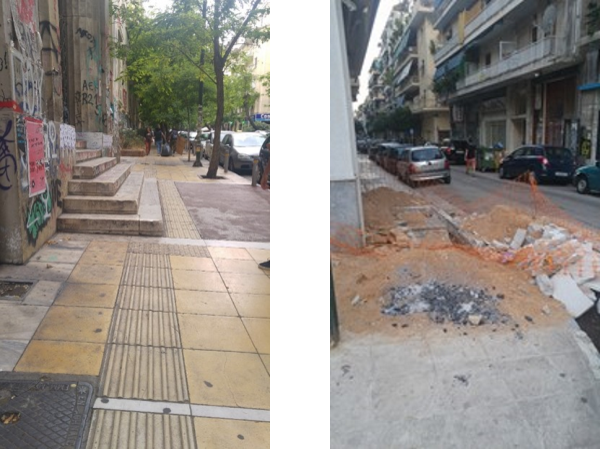Figure 7: Examples of not detected obstacles.